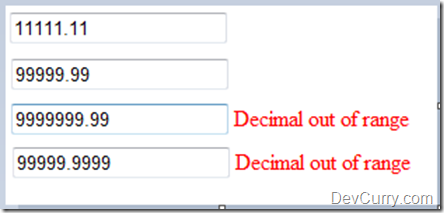# Using Regular Expression to Validate a Decimal Number

 Tweet

One of my clients had a requirement where a decimal input could not exceed 5,2 characters. In other words, the integral part of the decimal could not exceed more than 5 digits and the fractional part could not exceed more than 2 digits.

Eg: 99999.22 where 99999 is integral part and 22 is fractional

Here’s how to solve this requirement using a Regular expression:

`<asp:TextBox ID="txtN" runat="server"></asp:TextBox><asp:RegularExpressionValidator id="myRegex" runat="server"ControlToValidate="txtN" ValidationExpression="^[0-9]{1,5}(\.[0-9]{0,2})?\$"ErrorMessage="Decimal out of range" />`

The expression ^[0-9]{1,5}(\.[0-9]{0,2})?\$ checks if the integral part is between 1 to 5 digits and the fractional part is between 0 to 2 digits. Here are some tests.